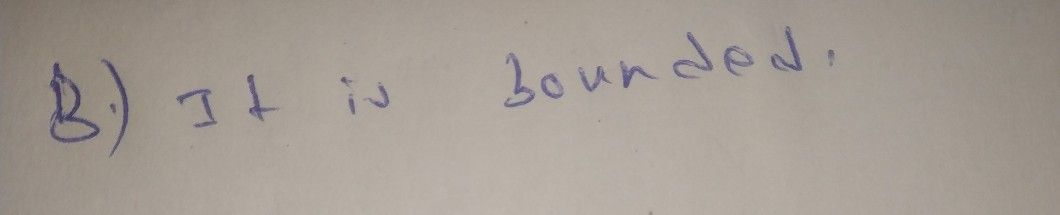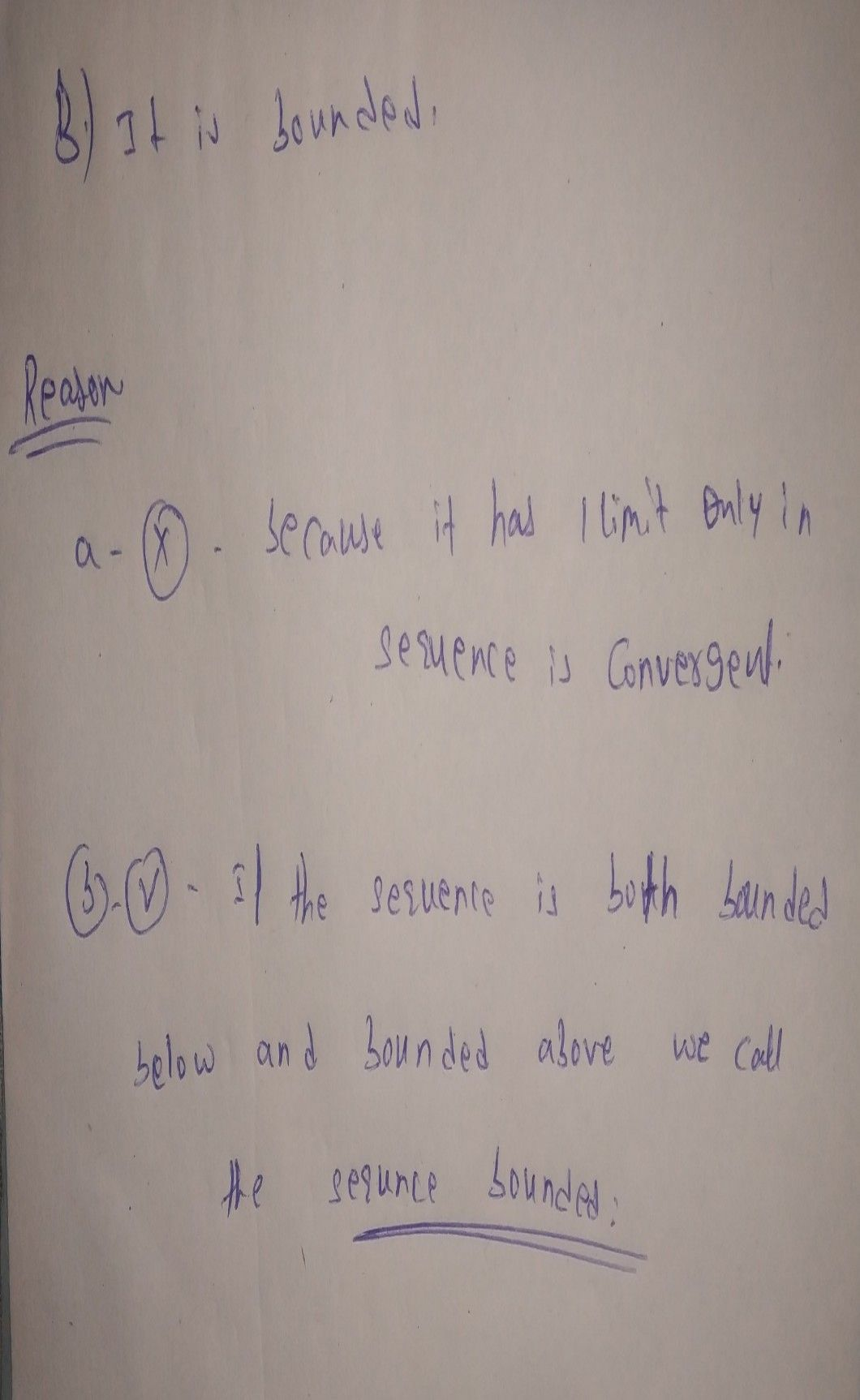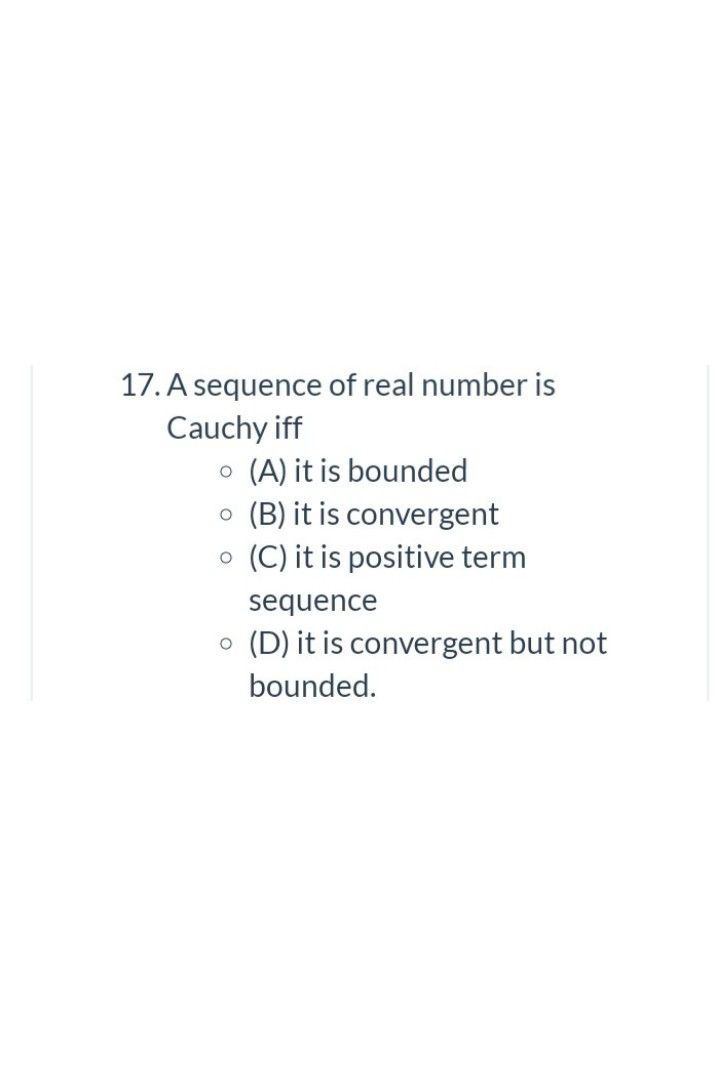Symbol
Problem$12.$ If the sequence is convergent then $O$ $1$ $A\right)$ it has two limits. $O$ $\left($ $B\right)$ it is bounded. $O$ $1$ $C\right)$ it is bounded above but may not be bounded below. $O$ o $\left($ (D) it is bounded below but may not be bounded above.
Other
Search count: 105
SolutionQanda teacher - k Ganeshwaitfeel free to ask me if you have any doubts related to the answerStudent
thank U sirQanda teacher - k Ganesh
give me good feedback with rating pleaseStudentQanda teacher - k Ganesh
option c is correct
give me good feedback and rattling please